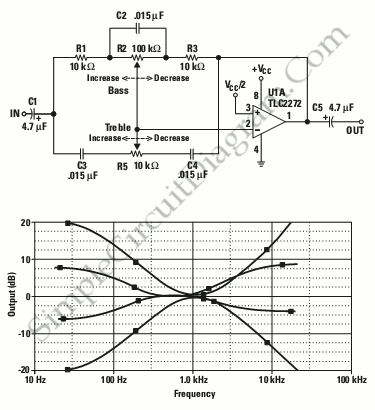# Single Op-Amp Tone Control

This is single op-amp tone control circuit. This circuit is a hybrid low-pass, high pass and one-pole circuits with attenuation and gain. This circuit is the solution for limits cost because it requires a minimum component. Here is the circuit :For the tone adjustments, the midrange frequency is 1 kHz. It gives about ±20 dB of  cut and boost for treble and bass. Unlike other similar circuits, it uses linear instead of logarithmic pots. This circuit uses two different potentiometer values and same value capacitors except for the coupling capacitor. The ideal capacitor value is 0.016 µF, which is an E-24 value. So the more common E-12 value of 0.015 µF is used instead. It is easier to find an adball capacitor value than an oddball potentiometer value. You can use a passive (resistors) voltage divider for VCC/2 or you can use an active  virtual ground circuit.

The second figure is a plots that show the response of he circuit with the pots at 1/4 and 3/4 and the extremes positions. The middle position is flat to within a few millidecibels. The pots are most sensitive towards the end of their travel, it was shown by the 1/4 and 3/4 positions are not exactly 10 and –10 dB. It gives more rapid adjustment near the extreme positions and a fine adjustment near the middle of potentiometers, which preferable to the listener. [Source: Analog Application Journal]